# Pythagorean theorem - math word problems

1. Square side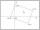Calculate length of side square ABCD with vertex A[0, 0] if diagonal BD lies on line p: -4x -5 =0.
2. Horizon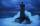The top of a lighthouse is 19 m above the sea. How far away is an object which is just “on the horizon”? [Assume the earth is a sphere of radius 6378.1 km.]
3. Elevation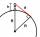What must be the elevation of an observer in order that he may be able to see an object on the earth 536 km away? Assume the earth to be a smooth sphere with radius 6378.1 km.
4. Center of the cubeCenter of the cube has distance 16 cm from each vertex. Calculate the volume V and surface area S of the cube.
5. Euklid4Legs of a right triangle have dimensions 244 m and 246 m. Calculate the length of the hypotenuse and the height of this right triangle.
6. RectangleCalculate the length of the side GN and diagonal QN of rectangle QGNH when given: |HN| = 25 cm and angle ∠ QGH = 28 degrees.
7. Unit vector 2DDetermine coordinates of unit vector to vector AB if A[-6; 8], B[-18; 10].
8. Column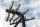Perpendicular pole high 8 m tall broke and its toe fell 2.7 m from the bottom of the pole. At what height above the ground pole broke?
9. RhombusThe rhombus with area 68 has one diagonal is longer by 6 than second one. Calculate the length of the diagonals and rhombus sides.
10. Circle chordDetermine the radius of the circle in which the chord 6 cm away from the center of the circle is 12 cm longer than the radius of the circle.
11. Similarity coefficientThe ratio of similarity of two equilateral triangles is 3.5 (ie 7:2). The length of the side of smaller triangle is 2.4 cm. Calculate the perimeter and area of ​​the larger triangle.
12. RhombusCalculate the length of the diagonal AC of the rhombus ABCD, if its perimeter is 84 dm and the other diagonal BD has length 20 dm.
13. Isosceles IIIThe base of the isosceles triangle is 17 cm area 416 cm2. Calculate the perimeter of this triangle.
14. Leg and heightSolve right triangle with height v = 9.6 m and shorter cathetus b = 17.3 m.
15. Without Euclid lawsRight triangle ABC with right angle at the C has a=5 and hypotenuse c=19. Calculate the height h of this triangle without the use of Euclidean laws.
16. Goniometric form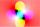Determine goniometric form of a complex number ?.
17. Cut and coneCalculate the volume of the rotation cone which lateral surface is circle arc with radius 15 cm and central angle 63 degrees.
18. Diagonals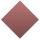Rhombus has two diagonals e=14 dm and f=11 dm. Calculate the side angle and height of the rhombus.
19. Oil rigOil drilling rig is 23 meters height and fix the ropes which ends are 7 meters away from the foot of the tower. How long are these ropes?
20. CanopyMr Peter has metal roof cone shape with a height of 127 cm and radius 130 cm over well. He needs paint the roof with anticorrosion. How many kg of color must he buy if the manufacturer specifies the consumption of 1 kg to 3.3 m2?

Do you have an interesting mathematical word problem that you can't solve it? Submit math problem, and we can try to solve it.

We will send a solution to your e-mail address. Solved examples are also published here. Please enter the e-mail correctly and check whether you don't have a full mailbox.

Please do not submit problems from current active competitions such as Mathematical Olympiad, correspondence seminars etc...

Pythagorean theorem is the base for the right triangle calculator.